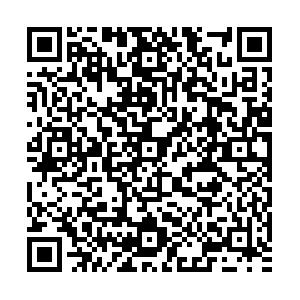# ${ \Xi^- t}$quasibound state instead of ΛΛnn bound state

• The coupled $\Lambda\Lambda nn-\Xi^- pnn$system was studied to investigate whether the inclusion of channel coupling is able to bind the $\Lambda\Lambda nn$system. We use a separable potential three-body model of the coupled $\Lambda\Lambda nn - \Xi^- pnn$system and a variational four-body calculation with realistic interactions. Our results exclude the possibility of a $\Lambda\Lambda nn$bound state by a large margin. Instead, we found a $\Xi^- t$quasibound state above the $\Lambda\Lambda nn$threshold.
••Tables(9)

Get Citation
H. Garcilazo, A. Valcarce and J. Vijande. ${ \Xi^- t}$quasibound state instead of ΛΛnn bound state[J]. Chinese Physics C, 2020, 44(2): 024102. doi: 10.1088/1674-1137/44/2/024102
H. Garcilazo, A. Valcarce and J. Vijande. ${ \Xi^- t}$quasibound state instead of ΛΛnn bound state[J]. Chinese Physics C, 2020, 44(2): 024102.Milestone
Received: 2019-10-04
Article Metric

Article Views(549)
PDF Downloads(31)
Cited by(0)
Policy on re-use
To reuse of subscription content published by CPC, the users need to request permission from CPC, unless the content was published under an Open Access license which automatically permits that type of reuse.
###### 通讯作者: 陈斌, bchen63@163.com
• 1.

沈阳化工大学材料科学与工程学院 沈阳 110142

Title:
Email:

## ${ \Xi^- t}$quasibound state instead of ΛΛnn bound state

###### Corresponding author: J. Vijande, javier.vijande@uv.es
• 1. Escuela Superior de Física y Matemáticas, Instituto Politécnico Nacional, Edificio 9, 07738 México D.F., Mexico
• 2. Departamento de Física Fundamental, Universidad de Salamanca, 37008 Salamanca, Spain
• 3. Departamento de Física Atómica, Molecular y Nuclear, Universidad de Valencia (UV) and IFIC (UV-CSIC), 46100 Valencia, Spain
• 4. IRIMED Joint Research Unit (IIS La Fe - UV), 46100 Valencia, Spain

Abstract: The coupled $\Lambda\Lambda nn-\Xi^- pnn$system was studied to investigate whether the inclusion of channel coupling is able to bind the $\Lambda\Lambda nn$system. We use a separable potential three-body model of the coupled $\Lambda\Lambda nn - \Xi^- pnn$system and a variational four-body calculation with realistic interactions. Our results exclude the possibility of a $\Lambda\Lambda nn$bound state by a large margin. Instead, we found a $\Xi^- t$quasibound state above the $\Lambda\Lambda nn$threshold.

### HTML2.   The ${\Lambda\Lambda (nn) - {\Xi^-} p (nn) }$ three-body model3.   The ${\Lambda\Lambda nn }$ and ${{ \Xi^-}pnn}$ four-body problems
Reference (34)
PDF查看关注分享

Top

### 目录/DownLoad:  Full-Size Img  PowerPoint